# Tag: permutation representation

While the verdict on a neolithic Scottish icosahedron is still open, let us recall Kostant’s group-theoretic construction of the icosahedron from its rotation-symmetry group $A_5$.The alternating group $A_5$ has two conjugacy classes of order 5 elements, both consisting of exactly 12 elements. Fix one of these conjugacy classes, say $C$ and construct a graph with vertices the 12 elements of $C$ and an edge between two $u,v \in C$ if and only if the group-product $u.v \in C$ still belongs to the same conjugacy class.

Observe that this relation is symmetric as from $u.v = w \in C$ it follows that $v.u=u^{-1}.u.v.u = u^{-1}.w.u \in C$. The graph obtained is the icosahedron, depicted on the right with vertices written as words in two adjacent elements u and v from $C$, as indicated.

Kostant writes : “Normally it is not a common practice in group theory to consider whether or not the product of two elements in a conjugacy class is again an element in that conjugacy class. However such a consideration here turns out to be quite productive.”

Still, similar constructions have been used in other groups as well, in particular in the study of the largest sporadic group, the monster group $\mathbb{M}$.

There is one important catch. Whereas it is quite trivial to multiply two permutations and verify whether the result is among 12 given ones, for most of us mortals it is impossible to do actual calculations in the monster. So, we’d better have an alternative way to get at the icosahedral graph using only $A_5$-data that is also available for the monster group, such as its character table.

Let $G$ be any finite group and consider three of its conjugacy classes $C(i),C(j)$ and $C(k)$. For any element $w \in C(k)$ we can compute from the character table of $G$ the number of different products $u.v = w$ such that $u \in C(i)$ and $v \in C(j)$. This number is given by the formula

$\frac{|G|}{|C_G(g_i)||C_G(g_j)|} \sum_{\chi} \frac{\chi(g_i) \chi(g_j) \overline{\chi(g_k)}}{\chi(1)}$

where the sum is taken over all irreducible characters $\chi$ and where $g_i \in C(i),g_j \in C(j)$ and $g_k \in C(k)$. Note also that $|C_G(g)|$ is the number of $G$-elements commuting with $g$ and that this number is the order of $G$ divided by the number of elements in the conjugacy class of $g$.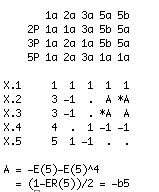The character table of $A_5$ is given on the left : the five columns correspond to the different conjugacy classes of elements of order resp. 1,2,3,5 and 5 and the rows are the character functions of the 5 irreducible representations of dimensions 1,3,3,4 and 5.

Let us fix the 4th conjugacy class, that is 5a, as our class $C$. By the general formula, for a fixed $w \in C$ the number of different products $u.v=w$ with $u,v \in C$ is equal to

$\frac{60}{25}(\frac{1}{1} + \frac{(\frac{1+\sqrt{5}}{2})^3}{3} + \frac{(\frac{1-\sqrt{5}}{2})^3}{3} – \frac{1}{4} + \frac{0}{5}) = \frac{60}{25}(1 + \frac{4}{3} – \frac{1}{4}) = 5$

Because for each $x \in C$ also its inverse $x^{-1} \in C$, this can be rephrased by saying that there are exactly 5 different products $w^{-1}.u \in C$, or equivalently, that the valency of every vertex $w^{-1} \in C$ in the graph is exactly 5.

That is, our graph has 12 vertices, each with exactly 5 neighbors, and with a bit of extra work one can show it to be the icosahedral graph.

For the monster group, the Atlas tells us that it has exactly 194 irreducible representations (and hence also 194 conjugacy classes). Of these conjugacy classes, the involutions (that is the elements of order 2) are of particular importance.There are exactly 2 conjugacy classes of involutions, usually denoted 2A and 2B. Involutions in class 2A are called “Fischer-involutions”, after Bernd Fischer, because their centralizer subgroup is an extension of Fischer’s baby Monster sporadic group.

Likewise, involutions in class 2B are usually called “Conway-involutions” because their centralizer subgroup is an extension of the largest Conway sporadic group.

Let us define the monster graph to be the graph having as its vertices the Fischer-involutions and with an edge between two of them $u,v \in 2A$ if and only if their product $u.v$ is again a Fischer-involution.

Because the centralizer subgroup is $2.\mathbb{B}$, the number of vertices is equal to $97239461142009186000 = 2^4 * 3^7 * 5^3 * 7^4 * 11 * 13^2 * 29 * 41 * 59 * 71$.

From the general result recalled before we have that the valency in all vertices is equal and to determine it we have to use the character table of the monster and the formula. Fortunately GAP provides the function ClassMultiplicationCoefficient to do this without making errors.

 gap> table:=CharacterTable("M"); CharacterTable( "M" ) gap> ClassMultiplicationCoefficient(table,2,2,2); 27143910000 

Perhaps noticeable is the fact that the prime decomposition of the valency $27143910000 = 2^4 * 3^4 * 5^4 * 23 * 31 * 47$ is symmetric in the three smallest and three largest prime factors of the baby monster order.

Robert Griess proved that one can recover the monster group $\mathbb{M}$ from the monster graph as its automorphism group!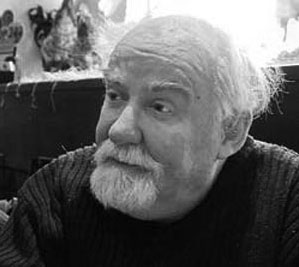As in the case of the icosahedral graph, the number of vertices and their common valency does not determine the monster graph uniquely. To gain more insight, we would like to know more about the sizes of minimal circuits in the graph, the number of such minimal circuits going through a fixed vertex, and so on.

Such an investigation quickly leads to a careful analysis which other elements can be obtained from products $u.v$ of two Fischer involutions $u,v \in 2A$. We are in for a major surprise, first observed by John McKay:

Printing out the number of products of two Fischer-involutions giving an element in the i-th conjugacy class of the monster,
where i runs over all 194 possible classes, we get the following string of numbers :

 97239461142009186000, 27143910000, 196560, 920808, 0, 3, 1104, 4, 0, 0, 5, 0, 6, 0, 0, 0, 0, 0, 0, 0, 0, 0, 0, 0, 0, 0, 0, 0, 0, 0, 0, 0, 0, 0, 0, 0, 0, 0, 0, 0, 0, 0, 0, 0, 0, 0, 0, 0, 0, 0, 0, 0, 0, 0, 0, 0, 0, 0, 0, 0, 0, 0, 0, 0, 0, 0, 0, 0, 0, 0, 0, 0, 0, 0, 0, 0, 0, 0, 0, 0, 0, 0, 0, 0, 0, 0, 0, 0, 0, 0, 0, 0, 0, 0, 0, 0, 0, 0, 0, 0, 0, 0, 0, 0, 0, 0, 0, 0, 0, 0, 0, 0, 0, 0, 0, 0, 0, 0, 0, 0, 0, 0, 0, 0, 0, 0, 0, 0, 0, 0, 0, 0, 0, 0, 0, 0, 0, 0, 0, 0, 0, 0, 0, 0, 0, 0, 0, 0, 0, 0, 0, 0, 0, 0, 0, 0, 0, 0, 0, 0, 0, 0, 0, 0, 0, 0, 0, 0, 0, 0, 0, 0, 0, 0, 0, 0, 0, 0, 0, 0, 0, 0, 0, 0, 0, 0, 0, 0, 0, 0, 0, 0, 0, 0 

That is, the elements of only 9 conjugacy classes can be written as products of two Fischer-involutions! These classes are :

• 1A = { 1 } written in 97239461142009186000 different ways (after all involutions have order two)
• 2A, each element of which can be written in exactly 27143910000 different ways (the valency)
• 2B, each element of which can be written in exactly 196560 different ways. Observe that this is the kissing number of the Leech lattice leading to a permutation representation of $2.Co_1$.
• 3A, each element of which can be written in exactly 920808 ways. Note that this number gives a permutation representation of the maximal monster subgroup $3.Fi_{24}’$.
• 3C, each element of which can be written in exactly 3 ways.
• 4A, each element of which can be written in exactly 1104 ways.
• 4B, each element of which can be written in exactly 4 ways.
• 5A, each element of which can be written in exactly 5 ways.
• 6A, each element of which can be written in exactly 6 ways.

Let us forget about the actual numbers for the moment and concentrate on the orders of these 9 conjugacy classes : 1,2,2,3,3,4,4,5,6. These are precisely the components of the fundamental root of the extended Dynkin diagram $\tilde{E_8}$!

This is the content of John McKay’s E(8)-observation : there should be a precise relation between the nodes of the extended Dynkin diagram and these 9 conjugacy classes in such a way that the order of the class corresponds to the component of the fundamental root. More precisely, one conjectures the following correspondence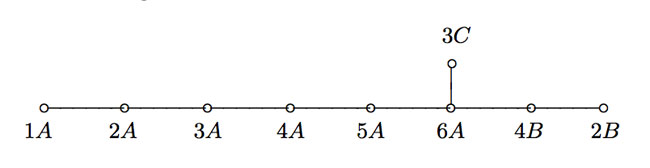This is similar to the classical McKay correspondence between finite subgroups of $SU(2)$ and extended Dynkin diagrams (the binary icosahedral group corresponding to extended E(8)). In that correspondence, the nodes of the Dynkin diagram correspond to irreducible representations of the group and the edges are determined by the decompositions of tensor-products with the fundamental 2-dimensional representation.

Here, however, the nodes have to correspond to conjugacy classes (rather than representations) and we have to look for another procedure to arrive at the required edges! An exciting proposal has been put forward recently by John Duncan in his paper Arithmetic groups and the affine E8 Dynkin diagram.

It will take us a couple of posts to get there, but for now, let’s give the gist of it : monstrous moonshine gives a correspondence between conjugacy classes of the monster and certain arithmetic subgroups of $PSL_2(\mathbb{R})$ commensurable with the modular group $\Gamma = PSL_2(\mathbb{Z})$. The edges of the extended Dynkin E(8) diagram are then given by the configuration of the arithmetic groups corresponding to the indicated 9 conjugacy classes! (to be continued…)

Last time we tried to generalize the Connes-Consani approach to commutative algebraic geometry over the field with one element $\mathbb{F}_1$ to the noncommutative world by considering covariant functors

$N~:~\mathbf{groups} \rightarrow \mathbf{sets}$

which over $\mathbb{C}$ resp. $\mathbb{Z}$ become visible by a complex (resp. integral) algebra having suitable universal properties.

However, we didn’t specify what we meant by a complex noncommutative variety (resp. an integral noncommutative scheme). In particular, we claimed that the $\mathbb{F}_1$-‘points’ associated to the functor

$D~:~\mathbf{groups} \rightarrow \mathbf{sets} \qquad G \mapsto G_2 \times G_3$ (here $G_n$ denotes all elements of order $n$ of $G$)

were precisely the modular dessins d’enfants of Grothendieck, but didn’t give details. We’ll try to do this now.

For algebras over a field we follow the definition, due to Kontsevich and Soibelman, of so called “noncommutative thin schemes”. Actually, the thinness-condition is implicit in both Soule’s-approach as that of Connes and Consani : we do not consider R-points in general, but only those of rings R which are finite and flat over our basering (or field).

So, what is a noncommutative thin scheme anyway? Well, its a covariant functor (commuting with finite projective limits)

$\mathbb{X}~:~\mathbf{Alg}^{fd}_k \rightarrow \mathbf{sets}$

from finite-dimensional (possibly noncommutative) $k$-algebras to sets. Now, the usual dual-space operator gives an anti-equivalence of categories

$\mathbf{Alg}^{fd}_k \leftrightarrow \mathbf{Coalg}^{fd}_k \qquad A=C^* \leftrightarrow C=A^*$

so a thin scheme can also be viewed as a contra-variant functor (commuting with finite direct limits)

$\mathbb{X}~:~\mathbf{Coalg}^{fd}_k \rightarrow \mathbf{Sets}$

In particular, we are interested to associated to any {tex]k $-algebra$A $its representation functor :$\mathbf{rep}(A)~:~\mathbf{Coalg}^{fd}_k \rightarrow \mathbf{Sets} \qquad C \mapsto Alg_k(A,C^*) $This may look strange at first sight, but$C^* $is a finite dimensional algebra and any$n $-dimensional representation of$A $is an algebra map$A \rightarrow M_n(k) $and we take$C $to be the dual coalgebra of this image. Kontsevich and Soibelman proved that every noncommutative thin scheme$\mathbb{X} $is representable by a$k $-coalgebra. That is, there exists a unique coalgebra$C_{\mathbb{X}} $(which they call the coalgebra of ‘distributions’ of$\mathbb{X} $) such that for every finite dimensional$k $-algebra$B $we have$\mathbb{X}(B) = Coalg_k(B^*,C_{\mathbb{X}}) $In the case of interest to us, that is for the functor$\mathbf{rep}(A) $the coalgebra of distributions is Kostant’s dual coalgebra$A^o $. This is the not the full linear dual of$A $but contains only those linear functionals on$A $which factor through a finite dimensional quotient. So? You’ve exchanged an algebra$A $for some coalgebra$A^o $, but where’s the geometry in all this? Well, let’s look at the commutative case. Suppose$A= \mathbb{C}[X] $is the coordinate ring of a smooth affine variety$X $, then its dual coalgebra looks like$\mathbb{C}[X]^o = \oplus_{x \in X} U(T_x(X)) $the direct sum of all universal (co)algebras of tangent spaces at points$x \in X $. But how do we get the variety out of this? Well, any coalgebra has a coradical (being the sun of all simple subcoalgebras) and in the case just mentioned we have$corad(\mathbb{C}[X]^o) = \oplus_{x \in X} \mathbb{C} e_x $so every point corresponds to a unique simple component of the coradical. In the general case, the coradical of the dual coalgebra$A^o $is the direct sum of all simple finite dimensional representations of$A $. That is, the direct summands of the coalgebra give us a noncommutative variety whose points are the simple representations, and the remainder of the coalgebra of distributions accounts for infinitesimal information on these points (as do the tangent spaces in the commutative case). In fact, it was a surprise to me that one can describe the dual coalgebra quite explicitly, and that$A_{\infty} $-structures make their appearance quite naturally. See this paper if you’re in for the details on this. That settles the problem of what we mean by the noncommutative variety associated to a complex algebra. But what about the integral case? In the above, we used extensively the theory of Kostant-duality which works only for algebras over fields… Well, not quite. In the case of$\mathbb{Z} $(or more general, of Dedekind domains) one can repeat Kostant’s proof word for word provided one takes as the definition of the dual$\mathbb{Z} $-coalgebra of an algebra (which is$\mathbb{Z} $-torsion free)$A^o = { f~:~A \rightarrow \mathbb{Z}~:~A/Ker(f)~\text{is finitely generated and torsion free}~} $(over general rings there may be also variants of this duality, as in Street’s book an Quantum groups). Probably lots of people have come up with this, but the only explicit reference I have is to the first paper I’ve ever written. So, also for algebras over$\mathbb{Z} $we can define a suitable noncommutative integral scheme (the coradical approach accounts only for the maximal ideals rather than all primes, but somehow this is implicit in all approaches as we consider only thin schemes). Fine! So, we can make sense of the noncommutative geometrical objects corresponding to the group-algebras$\mathbb{C} \Gamma $and$\mathbb{Z} \Gamma $where$\Gamma = PSL_2(\mathbb{Z}) $is the modular group (the algebras corresponding to the$G \mapsto G_2 \times G_3 $-functor). But, what might be the points of the noncommutative scheme corresponding to$\mathbb{F}_1 \Gamma $??? Well, let’s continue the path cut out before. “Points” should correspond to finite dimensional “simple representations”. Hence, what are the finite dimensional simple$\mathbb{F}_1 $-representations of$\Gamma $? (Or, for that matter, of any group$G $) Here we come back to Javier’s post on this : a finite dimensional$\mathbb{F}_1 $-vectorspace is a finite set. A$\Gamma $-representation on this set (of n-elements) is a group-morphism$\Gamma \rightarrow GL_n(\mathbb{F}_1) = S_n $hence it gives a permutation representation of$\Gamma $on this set. But then, if finite dimensional$\mathbb{F}_1 $-representations of$\Gamma $are the finite permutation representations, then the simple ones are the transitive permutation representations. That is, the points of the noncommutative scheme corresponding to$\mathbb{F}_1 \Gamma $are the conjugacy classes of subgroups$H \subset \Gamma $such that$\Gamma/H $is finite. But these are exactly the modular dessins d’enfants introduced by Grothendieck as I explained a while back elsewhere (see for example this post and others in the same series). The Monster is the largest of the 26 sporadic simple groups and has order 808 017 424 794 512 875 886 459 904 961 710 757 005 754 368 000 000 000 = 2^46 3^20 5^9 7^6 11^2 13^3 17 19 23 29 31 41 47 59 71. It is not so much the size of its order that makes it hard to do actual calculations in the monster, but rather the dimensions of its smallest non-trivial irreducible representations (196 883 for the smallest, 21 296 876 for the next one, and so on). In characteristic two there is an irreducible representation of one dimension less (196 882) which appears to be of great use to obtain information. For example, Robert Wilson used it to prove that The Monster is a Hurwitz group. This means that the Monster is generated by two elements g and h satisfying the relations$g^2 = h^3 = (gh)^7 = 1 $Geometrically, this implies that the Monster is the automorphism group of a Riemann surface of genus g satisfying the Hurwitz bound 84(g-1)=#Monster. That is, g=9619255057077534236743570297163223297687552000000001=42151199 * 293998543 * 776222682603828537142813968452830193 Or, in analogy with the Klein quartic which can be constructed from 24 heptagons in the tiling of the hyperbolic plane, there is a finite region of the hyperbolic plane, tiled with heptagons, from which we can construct this monster curve by gluing the boundary is a specific way so that we get a Riemann surface with exactly 9619255057077534236743570297163223297687552000000001 holes. This finite part of the hyperbolic tiling (consisting of #Monster/7 heptagons) we’ll call the empire of the monster and we’d love to describe it in more detail.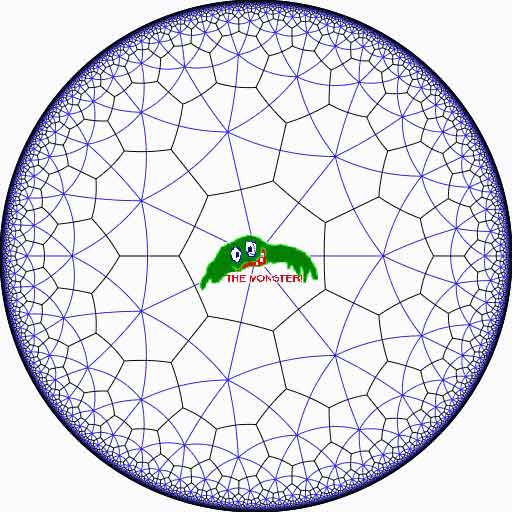Look at the half-edges of all the heptagons in the empire (the picture above learns that every edge in cut in two by a blue geodesic). They are exactly #Monster such half-edges and they form a dessin d’enfant for the monster-curve. If we label these half-edges by the elements of the Monster, then multiplication by g in the monster interchanges the two half-edges making up a heptagonal edge in the empire and multiplication by h in the monster takes a half-edge to the one encountered first by going counter-clockwise in the vertex of the heptagonal tiling. Because g and h generated the Monster, the dessin of the empire is just a concrete realization of the monster. Because g is of order two and h is of order three, the two permutations they determine on the dessin, gives a group epimorphism$C_2 \ast C_3 = PSL_2(\mathbb{Z}) \rightarrow \mathbb{M} $from the modular group$PSL_2(\mathbb{Z}) $onto the Monster-group. In noncommutative geometry, the group-algebra of the modular group$\mathbb{C} PSL_2 $can be interpreted as the coordinate ring of a noncommutative manifold (because it is formally smooth in the sense of Kontsevich-Rosenberg or Cuntz-Quillen) and the group-algebra of the Monster$\mathbb{C} \mathbb{M} $itself corresponds in this picture to a finite collection of ‘points’ on the manifold. Using this geometric viewpoint we can now ask the question What does the Monster see of the modular group? To make sense of this question, let us first consider the commutative equivalent : what does a point P see of a commutative variety X?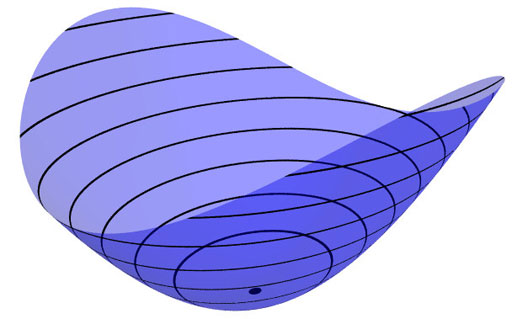Evaluation of polynomial functions in P gives us an algebra epimorphism$\mathbb{C}[X] \rightarrow \mathbb{C} $from the coordinate ring of the variety$\mathbb{C}[X] $onto$\mathbb{C} $and the kernel of this map is the maximal ideal$\mathfrak{m}_P $of$\mathbb{C}[X] $consisting of all functions vanishing in P. Equivalently, we can view the point$P= \mathbf{spec}~\mathbb{C}[X]/\mathfrak{m}_P $as the scheme corresponding to the quotient$\mathbb{C}[X]/\mathfrak{m}_P $. Call this the 0-th formal neighborhood of the point P. This sounds pretty useless, but let us now consider higher-order formal neighborhoods. Call the affine scheme$\mathbf{spec}~\mathbb{C}[X]/\mathfrak{m}_P^{n+1} $the n-th forml neighborhood of P, then the first neighborhood, that is with coordinate ring$\mathbb{C}[X]/\mathfrak{m}_P^2 $gives us tangent-information. Alternatively, it gives the best linear approximation of functions near P. The second neighborhood$\mathbb{C}[X]/\mathfrak{m}_P^3 $gives us the best quadratic approximation of function near P, etc. etc. These successive quotients by powers of the maximal ideal$\mathfrak{m}_P $form a system of algebra epimorphisms$\ldots \frac{\mathbb{C}[X]}{\mathfrak{m}_P^{n+1}} \rightarrow \frac{\mathbb{C}[X]}{\mathfrak{m}_P^{n}} \rightarrow \ldots \ldots \rightarrow \frac{\mathbb{C}[X]}{\mathfrak{m}_P^{2}} \rightarrow \frac{\mathbb{C}[X]}{\mathfrak{m}_P} = \mathbb{C} $and its inverse limit$\underset{\leftarrow}{lim}~\frac{\mathbb{C}[X]}{\mathfrak{m}_P^{n}} = \hat{\mathcal{O}}_{X,P} $is the completion of the local ring in P and contains all the infinitesimal information (to any order) of the variety X in a neighborhood of P. That is, this completion$\hat{\mathcal{O}}_{X,P} $contains all information that P can see of the variety X. In case P is a smooth point of X, then X is a manifold in a neighborhood of P and then this completion$\hat{\mathcal{O}}_{X,P} $is isomorphic to the algebra of formal power series$\mathbb{C}[[ x_1,x_2,\ldots,x_d ]] $where the$x_i $form a local system of coordinates for the manifold X near P. Right, after this lengthy recollection, back to our question what does the monster see of the modular group? Well, we have an algebra epimorphism$\pi~:~\mathbb{C} PSL_2(\mathbb{Z}) \rightarrow \mathbb{C} \mathbb{M} $and in analogy with the commutative case, all information the Monster can gain from the modular group is contained in the$\mathfrak{m} $-adic completion$\widehat{\mathbb{C} PSL_2(\mathbb{Z})}_{\mathfrak{m}} = \underset{\leftarrow}{lim}~\frac{\mathbb{C} PSL_2(\mathbb{Z})}{\mathfrak{m}^n} $where$\mathfrak{m} $is the kernel of the epimorphism$\pi $sending the two free generators of the modular group$PSL_2(\mathbb{Z}) = C_2 \ast C_3 $to the permutations g and h determined by the dessin of the pentagonal tiling of the Monster’s empire. As it is a hopeless task to determine the Monster-empire explicitly, it seems even more hopeless to determine the kernel$\mathfrak{m} $let alone the completed algebra… But, (surprise) we can compute$\widehat{\mathbb{C} PSL_2(\mathbb{Z})}_{\mathfrak{m}} $as explicitly as in the commutative case we have$\hat{\mathcal{O}}_{X,P} \simeq \mathbb{C}[[ x_1,x_2,\ldots,x_d ]] $for a point P on a manifold X. Here the details : the quotient$\mathfrak{m}/\mathfrak{m}^2 $has a natural structure of$\mathbb{C} \mathbb{M} $-bimodule. The group-algebra of the monster is a semi-simple algebra, that is, a direct sum of full matrix-algebras of sizes corresponding to the dimensions of the irreducible monster-representations. That is,$\mathbb{C} \mathbb{M} \simeq \mathbb{C} \oplus M_{196883}(\mathbb{C}) \oplus M_{21296876}(\mathbb{C}) \oplus \ldots \ldots \oplus M_{258823477531055064045234375}(\mathbb{C}) $with exactly 194 components (the number of irreducible Monster-representations). For any$\mathbb{C} \mathbb{M} $-bimodule$M $one can form the tensor-algebra$T_{\mathbb{C} \mathbb{M}}(M) = \mathbb{C} \mathbb{M} \oplus M \oplus (M \otimes_{\mathbb{C} \mathbb{M}} M) \oplus (M \otimes_{\mathbb{C} \mathbb{M}} M \otimes_{\mathbb{C} \mathbb{M}} M) \oplus \ldots \ldots $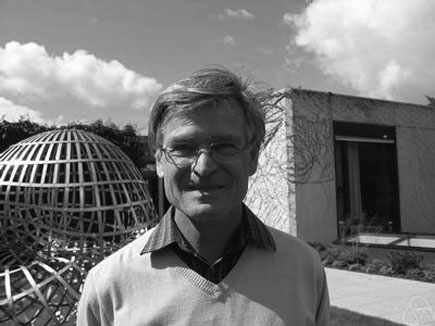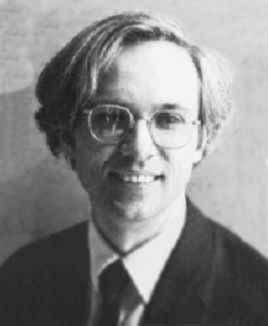and applying the formal neighborhood theorem for formally smooth algebras (such as$\mathbb{C} PSL_2(\mathbb{Z}) $) due to Joachim Cuntz (left) and Daniel Quillen (right) we have an isomorphism of algebras$\widehat{\mathbb{C} PSL_2(\mathbb{Z})}_{\mathfrak{m}} \simeq \widehat{T_{\mathbb{C} \mathbb{M}}(\mathfrak{m}/\mathfrak{m}^2)} $where the right-hand side is the completion of the tensor-algebra (at the unique graded maximal ideal) of the$\mathbb{C} \mathbb{M} $-bimodule$\mathfrak{m}/\mathfrak{m}^2 $, so we’d better describe this bimodule explicitly. Okay, so what’s a bimodule over a semisimple algebra of the form$S=M_{n_1}(\mathbb{C}) \oplus \ldots \oplus M_{n_k}(\mathbb{C}) $? Well, a simple S-bimodule must be either (1) a factor$M_{n_i}(\mathbb{C}) $with all other factors acting trivially or (2) the full space of rectangular matrices$M_{n_i \times n_j}(\mathbb{C}) $with the factor$M_{n_i}(\mathbb{C}) $acting on the left,$M_{n_j}(\mathbb{C}) $acting on the right and all other factors acting trivially. That is, any S-bimodule can be represented by a quiver (that is a directed graph) on k vertices (the number of matrix components) with a loop in vertex i corresponding to each simple factor of type (1) and a directed arrow from i to j corresponding to every simple factor of type (2). That is, for the Monster, the bimodule$\mathfrak{m}/\mathfrak{m}^2 $is represented by a quiver on 194 vertices and now we only have to determine how many loops and arrows there are at or between vertices. Using Morita equivalences and standard representation theory of quivers it isn’t exactly rocket science to determine that the number of arrows between the vertices corresponding to the irreducible Monster-representations$S_i $and$S_j $is equal to$dim_{\mathbb{C}}~Ext^1_{\mathbb{C} PSL_2(\mathbb{Z})}(S_i,S_j)-\delta_{ij} $Now, I’ve been wasting a lot of time already here explaining what representations of the modular group have to do with quivers (see for example here or some other posts in the same series) and for quiver-representations we all know how to compute Ext-dimensions in terms of the Euler-form applied to the dimension vectors. Right, so for every Monster-irreducible$S_i $we have to determine the corresponding dimension-vector$~(a_1,a_2;b_1,b_2,b_3) $for the quiver$\xymatrix{ & & & &
\vtx{b_1} \\ \vtx{a_1} \ar[rrrru]^(.3){B_{11}} \ar[rrrrd]^(.3){B_{21}}
\ar[rrrrddd]_(.2){B_{31}} & & & & \\ & & & & \vtx{b_2} \\ \vtx{a_2}
\ar[rrrruuu]_(.7){B_{12}} \ar[rrrru]_(.7){B_{22}}
\ar[rrrrd]_(.7){B_{23}} & & & & \\ & & & & \vtx{b_3}} $Now the dimensions$a_i $are the dimensions of the +/-1 eigenspaces for the order 2 element g in the representation and the$b_i $are the dimensions of the eigenspaces for the order 3 element h. So, we have to determine to which conjugacy classes g and h belong, and from Wilson’s paper mentioned above these are classes 2B and 3B in standard Atlas notation. So, for each of the 194 irreducible Monster-representations we look up the character values at 2B and 3B (see below for the first batch of those) and these together with the dimensions determine the dimension vector$~(a_1,a_2;b_1,b_2,b_3) $.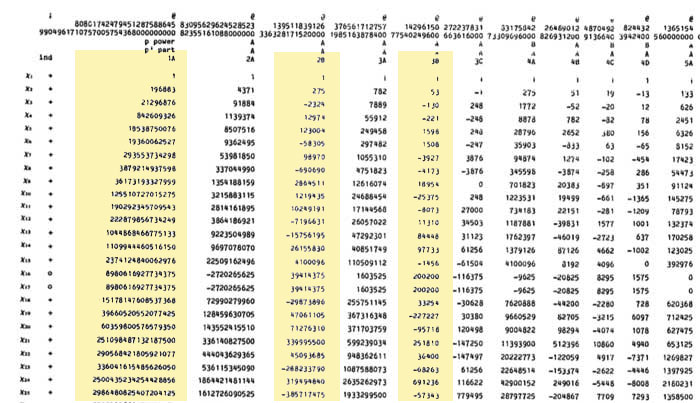For example take the 196883-dimensional irreducible. Its 2B-character is 275 and the 3B-character is 53. So we are looking for a dimension vector such that$a_1+a_2=196883, a_1-275=a_2 $and$b_1+b_2+b_3=196883, b_1-53=b_2=b_3 $giving us for that representation the dimension vector of the quiver above$~(98579,98304,65663,65610,65610) $. Okay, so for each of the 194 irreducibles$S_i $we have determined a dimension vector$~(a_1(i),a_2(i);b_1(i),b_2(i),b_3(i)) $, then standard quiver-representation theory asserts that the number of loops in the vertex corresponding to$S_i $is equal to$dim(S_i)^2 + 1 – a_1(i)^2-a_2(i)^2-b_1(i)^2-b_2(i)^2-b_3(i)^2 $and that the number of arrows from vertex$S_i $to vertex$S_j $is equal to$dim(S_i)dim(S_j) – a_1(i)a_1(j)-a_2(i)a_2(j)-b_1(i)b_1(j)-b_2(i)b_2(j)-b_3(i)b_3(j) $This data then determines completely the$\mathbb{C} \mathbb{M} $-bimodule$\mathfrak{m}/\mathfrak{m}^2 $and hence the structure of the completion$\widehat{\mathbb{C} PSL_2}_{\mathfrak{m}} $containing all information the Monster can gain from the modular group. But then, one doesn’t have to go for the full regular representation of the Monster. Any faithful permutation representation will do, so we might as well go for the one of minimal dimension. That one is known to correspond to the largest maximal subgroup of the Monster which is known to be a two-fold extension$2.\mathbb{B} $of the Baby-Monster. The corresponding permutation representation is of dimension 97239461142009186000 and decomposes into Monster-irreducibles$S_1 \oplus S_2 \oplus S_4 \oplus S_5 \oplus S_9 \oplus S_{14} \oplus S_{21} \oplus S_{34} \oplus S_{35} $(in standard Atlas-ordering) and hence repeating the arguments above we get a quiver on just 9 vertices! The actual numbers of loops and arrows (I forgot to mention this, but the quivers obtained are actually symmetric) obtained were found after laborious computations mentioned in this post and the details I’ll make avalable here. Anyone who can spot a relation between the numbers obtained and any other part of mathematics will obtain quantities of genuine (ie. non-Inbev) Belgian beer… “Ne pleure pas, Alfred ! J’ai besoin de tout mon courage pour mourir à vingt ans!” We all remember the last words of Evariste Galois to his brother Alfred. Lesser known are the mathematical results contained in his last letter, written to his friend Auguste Chevalier, on the eve of his fatal duel. Here the final sentences :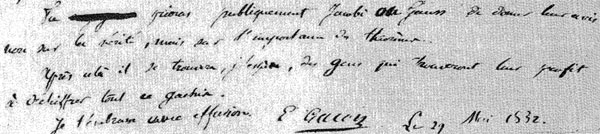Tu prieras publiquement Jacobi ou Gauss de donner leur avis non sur la verite, mais sur l’importance des theoremes. Apres cela il se trouvera, j’espere, des gens qui trouvent leur profis a dechiffrer tout ce gachis. Je t’embrasse avec effusion. E. Galois, le 29 Mai 1832 A major result contained in this letter concerns the groups$L_2(p)=PSL_2(\mathbb{F}_p) $, that is the group of$2 \times 2 $matrices with determinant equal to one over the finite field$\mathbb{F}_p $modulo its center.$L_2(p) $is known to be simple whenever$p \geq 5 $. Galois writes that$L_2(p) $cannot have a non-trivial permutation representation on fewer than$p+1 $symbols whenever$p > 11 $and indicates the transitive permutation representation on exactly$p $symbols in the three ‘exceptional’ cases$p=5,7,11 $. Let$\alpha = \begin{bmatrix} 1 & 1 \\ 0 & 1 \end{bmatrix} $and consider for$p=5,7,11 $the involutions on$\mathbb{P}^1_{\mathbb{F}_p} = \mathbb{F}_p \cup { \infty } $(on which$L_2(p) $acts via Moebius transformations)$\pi_5 = (0,\infty)(1,4)(2,3) \quad \pi_7=(0,\infty)(1,3)(2,6)(4,5) \quad \pi_{11}=(0,\infty)(1,6)(3,7)(9,10)(5,8)(4,2) $(in fact, Galois uses the involution$~(0,\infty)(1,2)(3,6)(4,8)(5,10)(9,7) $for$p=11 $), then$L_2(p) $leaves invariant the set consisting of the$p $involutions$\Pi = { \alpha^{-i} \pi_p \alpha^i~:~1 \leq i \leq p } $. After mentioning these involutions Galois merely writes : Ainsi pour le cas de$p=5,7,11 $, l’equation modulaire s’abaisse au degre p. En toute rigueur, cette reduction n’est pas possible dans les cas plus eleves. Alternatively, one can deduce these permutation representation representations from group isomorphisms. As$L_2(5) \simeq A_5 $, the alternating group on 5 symbols,$L_2(5) $clearly acts transitively on 5 symbols.Similarly, for$p=7 $we have$L_2(7) \simeq L_3(2) $and so the group acts as automorphisms on the projective plane over the field on two elements$\mathbb{P}^2_{\mathbb{F}_2} $aka the Fano plane, as depicted on the left. This finite projective plane has 7 points and 7 lines and$L_3(2) $acts transitively on them. For$p=11 $the geometrical object is a bit more involved. The set of non-squares in$\mathbb{F}_{11} $is${ 1,3,4,5,9 } $and if we translate this set using the additive structure in$\mathbb{F}_{11} $one obtains the following 11 five-element sets${ 1,3,4,5,9 }, { 2,4,5,6,10 }, { 3,5,6,7,11 }, { 1,4,6,7,8 }, { 2,5,7,8,9 }, { 3,6,8,9,10 },  { 4,7,9,10,11 }, { 1,5,8,10,11 }, { 1,2,6,9,11 }, { 1,2,3,7,10 }, { 2,3,4,8,11 } $and if we regard these sets as ‘lines’ we see that two distinct lines intersect in exactly 2 points and that any two distinct points lie on exactly two ‘lines’. That is, intersection sets up a bijection between the 55-element set of all pairs of distinct points and the 55-element set of all pairs of distinct ‘lines’. This is called the biplane geometry. The subgroup of$S_{11} $(acting on the eleven elements of$\mathbb{F}_{11} $) stabilizing this set of 11 5-element sets is precisely the group$L_2(11) $giving the permutation representation on 11 objects. An alternative statement of Galois’ result is that for$p > 11 $there is no subgroup of$L_2(p) $complementary to the cyclic subgroup$C_p = { \begin{bmatrix} 1 & x \\ 0 & 1 \end{bmatrix}~:~x \in \mathbb{F}_p } $That is, there is no subgroup such that set-theoretically$L_2(p) = F \times C_p $(note this is of courese not a group-product, all it says is that any element can be written as$g=f.c $with$f \in F, c \in C_p $. However, in the three exceptional cases we do have complementary subgroups. In fact, set-theoretically we have$L_2(5) = A_4 \times C_5 \qquad L_2(7) = S_4 \times C_7 \qquad L_2(11) = A_5 \times C_{11} $and it is a truly amazing fact that the three groups appearing are precisely the three Platonic groups!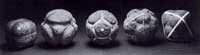Recall that here are 5 Platonic (or Scottish) solids coming in three sorts when it comes to rotation-automorphism groups : the tetrahedron (group$A_4 $), the cube and octahedron (group$S_4 $) and the dodecahedron and icosahedron (group$A_5 $). The “4” in the cube are the four body diagonals and the “5” in the dodecahedron are the five inscribed cubes. That is, our three ‘exceptional’ Galois-groups correspond to the three Platonic groups, which in turn correspond to the three exceptional Lie algebras$E_6,E_7,E_8 $via McKay correspondence (wrt. their 2-fold covers). Maybe I’ll detail this latter connection another time. It sure seems that surprises often come in triples… Finally, it is well known that$L_2(5) \simeq A_5 $is the automorphism group of the icosahedron (or dodecahedron) and that$L_2(7) $is the automorphism group of the Klein quartic. So, one might ask : is there also a nice curve connected with the third group$L_2(11) $? Rumour has it that this is indeed the case and that the curve in question has genus 70… (to be continued). Reference John Conway once wrote : There are almost as many different constructions of$M_{24} $as there have been mathematicians interested in that most remarkable of all finite groups. In the inguanodon post Ive added yet another construction of the Mathieu groups$M_{12} $and$M_{24} $starting from (half of) the Farey sequences and the associated cuboid tree diagram obtained by demanding that all edges are odd. In this way the Mathieu groups turned out to be part of a (conjecturally) infinite sequence of simple groups, starting as follows :$L_2(7),M_{12},A_{16},M_{24},A_{28},A_{40},A_{48},A_{60},A_{68},A_{88},A_{96},A_{120},A_{132},A_{148},A_{164},A_{196},\ldots $It is quite easy to show that none of the other sporadics will appear in this sequence via their known permutation representations. Still, several of the sporadic simple groups are generated by an element of order two and one of order three, so they are determined by a finite dimensional permutation representation of the modular group$PSL_2(\mathbb{Z}) $and hence are hiding in a special polygonal region of the Dedekind’s tessellation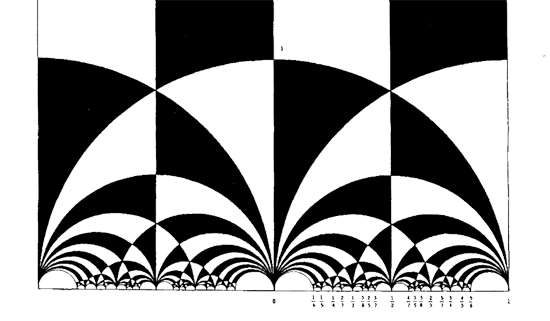Let us try to figure out where the sporadic with the next simplest permutation representation is hiding : the second Janko group$J_2 $, via its 100-dimensional permutation representation. The Atlas tells us that the order two and three generators act as e:= (1,84)(2,20)(3,48)(4,56)(5,82)(6,67)(7,55)(8,41)(9,35)(10,40)(11,78)(12, 100)(13,49)(14,37)(15,94)(16,76)(17,19)(18,44)(21,34)(22,85)(23,92)(24, 57)(25,75)(26,28)(27,64)(29,90)(30,97)(31,38)(32,68)(33,69)(36,53)(39,61) (42,73)(43,91)(45,86)(46,81)(47,89)(50,93)(51,96)(52,72)(54,74)(58,99) (59,95)(60,63)(62,83)(65,70)(66,88)(71,87)(77,98)(79,80); v:= (1,80,22)(2,9,11)(3,53,87)(4,23,78)(5,51,18)(6,37,24)(8,27,60)(10,62,47) (12,65,31)(13,64,19)(14,61,52)(15,98,25)(16,73,32)(17,39,33)(20,97,58) (21,96,67)(26,93,99)(28,57,35)(29,71,55)(30,69,45)(34,86,82)(38,59,94) (40,43,91)(42,68,44)(46,85,89)(48,76,90)(49,92,77)(50,66,88)(54,95,56) (63,74,72)(70,81,75)(79,100,83);  But as the kfarey.sage package written by Chris Kurth calculates the Farey symbol using the L-R generators, we use GAP to find those L = e*v^-1 and R=e*v^-2 so L=(1,84,22,46,70,12,79)(2,58,93,88,50,26,35)(3,90,55,7,71,53,36)(4,95,38,65,75,98,92)(5,86,69,39,14,6,96)(8,41,60,72,61,17, 64)(9,57,37,52,74,56,78)(10,91,40,47,85,80,83)(11,23,49,19,33,30,20)(13,77,15,59,54,63,27)(16,48,87,29,76,32,42)(18,68, 73,44,51,21,82)(24,28,99,97,45,34,67)(25,81,89,62,100,31,94) R=(1,84,80,100,65,81,85)(2,97,69,17,13,92,78)(3,76,73,68,16,90,71)(4,54,72,14,24,35,11)(5,34,96,18,42,32,44)(6,21,86,30,58, 26,57)(7,29,48,53,36,87,55)(8,41,27,19,39,52,63)(9,28,93,66,50,99,20)(10,43,40,62,79,22,89)(12,83,47,46,75,15,38)(23,77, 25,70,31,59,56)(33,45,82,51,67,37,61)(49,64,60,74,95,94,98)  Defining these permutations in sage and using kfarey, this gives us the Farey-symbol of the associated permutation representation L=SymmetricGroup(Integer(100))("(1,84,22,46,70,12,79)(2,58,93,88,50,26,35)(3,90,55,7,71,53,36)(4,95,38,65,75,98,92)(5,86,69,39,14,6,96)(8,41,60,72,61,17, 64)(9,57,37,52,74,56,78)(10,91,40,47,85,80,83)(11,23,49,19,33,30,20)(13,77,15,59,54,63,27)(16,48,87,29,76,32,42)(18,68, 73,44,51,21,82)(24,28,99,97,45,34,67)(25,81,89,62,100,31,94)") R=SymmetricGroup(Integer(100))("(1,84,80,100,65,81,85)(2,97,69,17,13,92,78)(3,76,73,68,16,90,71)(4,54,72,14,24,35,11)(5,34,96,18,42,32,44)(6,21,86,30,58, 26,57)(7,29,48,53,36,87,55)(8,41,27,19,39,52,63)(9,28,93,66,50,99,20)(10,43,40,62,79,22,89)(12,83,47,46,75,15,38)(23,77, 25,70,31,59,56)(33,45,82,51,67,37,61)(49,64,60,74,95,94,98)") sage: FareySymbol("Perm",[L,R]) [[0, 1, 4, 3, 2, 5, 18, 13, 21, 71, 121, 413, 292, 463, 171, 50, 29, 8, 27, 46, 65, 19, 30, 11, 3, 10, 37, 64, 27, 17, 7, 4, 5], [1, 1, 3, 2, 1, 2, 7, 5, 8, 27, 46, 157, 111, 176, 65, 19, 11, 3, 10, 17, 24, 7, 11, 4, 1, 3, 11, 19, 8, 5, 2, 1, 1], [-3, 1, 4, 4, 2, 3, 6, -3, 7, 13, 14, 15, -3, -3, 15, 14, 11, 8, 8, 10, 12, 12, 10, 9, 5, 5, 9, 11, 13, 7, 6, 3, 2, 1]]  Here, the first string gives the numerators of the cusps, the second the denominators and the third gives the pairing information (where [tex[-2$ denotes an even edge and $-3$ an odd edge. Fortunately, kfarey also allows us to draw the special polygonal region determined by a Farey-symbol. So, here it is (without the pairing data) :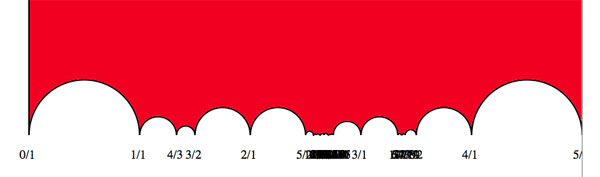the hiding place of $J_2$…

It would be nice to have (a) other Farey-symbols associated to the second Janko group, hopefully showing a pattern that one can extend into an infinite family as in the inguanodon series and (b) to determine Farey-symbols of more sporadic groups.

It’s been a while, so let’s include a recap : a (transitive) permutation representation of the modular group $\Gamma = PSL_2(\mathbb{Z})$ is determined by the conjugacy class of a cofinite subgroup $\Lambda \subset \Gamma$, or equivalently, to a dessin d’enfant. We have introduced a quiver (aka an oriented graph) which comes from a triangulation of the compactification of $\mathbb{H} / \Lambda$ where $\mathbb{H}$ is the hyperbolic upper half-plane. This quiver is independent of the chosen embedding of the dessin in the Dedeking tessellation. (For more on these terms and constructions, please consult the series Modular subgroups and Dessins d’enfants).

Why are quivers useful? To start, any quiver $Q$ defines a noncommutative algebra, the path algebra $\mathbb{C} Q$, which has as a $\mathbb{C}$-basis all oriented paths in the quiver and multiplication is induced by concatenation of paths (when possible, or zero otherwise). Usually, it is quite hard to make actual computations in noncommutative algebras, but in the case of path algebras you can just see what happens.

Moreover, we can also see the finite dimensional representations of this algebra $\mathbb{C} Q$. Up to isomorphism they are all of the following form : at each vertex $v_i$ of the quiver one places a finite dimensional vectorspace $\mathbb{C}^{d_i}$ and any arrow in the quiver
$$\xymatrix{\vtx{v_i} \ar[r]^a & \vtx{v_j}}$$ determines a linear map between these vertex spaces, that is, to $a$ corresponds a matrix in $M_{d_j \times d_i}(\mathbb{C})$. These matrices determine how the paths of length one act on the representation, longer paths act via multiplcation of matrices along the oriented path.

A necklace in the quiver is a closed oriented path in the quiver up to cyclic permutation of the arrows making up the cycle. That is, we are free to choose the start (and end) point of the cycle. For example, in the one-cycle quiver

$$\xymatrix{\vtx{} \ar[rr]^a & & \vtx{} \ar[ld]^b \\ & \vtx{} \ar[lu]^c &}$$

the basic necklace can be represented as $abc$ or $bca$ or $cab$. How does a necklace act on a representation? Well, the matrix-multiplication of the matrices corresponding to the arrows gives a square matrix in each of the vertices in the cycle. Though the dimensions of this matrix may vary from vertex to vertex, what does not change (and hence is a property of the necklace rather than of the particular choice of cycle) is the trace of this matrix. That is, necklaces give complex-valued functions on representations of $\mathbb{C} Q$ and by a result of Artin and Procesi there are enough of them to distinguish isoclasses of (semi)simple representations! That is, linear combinations a necklaces (aka super-potentials) can be viewed, after taking traces, as complex-valued functions on all representations (similar to character-functions).

In physics, one views these functions as potentials and it then interested in the points (representations) where this function is extremal (minimal) : the vacua. Clearly, this does not make much sense in the complex-case but is relevant when we look at the real-case (where we look at skew-Hermitian matrices rather than all matrices). A motivating example (the Yang-Mills potential) is given in Example 2.3.2 of Victor Ginzburg’s paper Calabi-Yau algebras.

Let $\Phi$ be a super-potential (again, a linear combination of necklaces) then our commutative intuition tells us that extrema correspond to zeroes of all partial differentials $\frac{\partial \Phi}{\partial a}$ where $a$ runs over all coordinates (in our case, the arrows of the quiver). One can make sense of differentials of necklaces (and super-potentials) as follows : the partial differential with respect to an arrow $a$ occurring in a term of $\Phi$ is defined to be the path in the quiver one obtains by removing all 1-occurrences of $a$ in the necklaces (defining $\Phi$) and rearranging terms to get a maximal broken necklace (using the cyclic property of necklaces). An example, for the cyclic quiver above let us take as super-potential $abcabc$ (2 cyclic turns), then for example

$\frac{\partial \Phi}{\partial b} = cabca+cabca = 2 cabca$

(the first term corresponds to the first occurrence of $b$, the second to the second). Okay, but then the vacua-representations will be the representations of the quotient-algebra (which I like to call the vacualgebra)

$\mathcal{U}(Q,\Phi) = \frac{\mathbb{C} Q}{(\partial \Phi/\partial a, \forall a)}$

which in ‘physical relevant settings’ (whatever that means…) turn out to be Calabi-Yau algebras.

But, let us return to the case of subgroups of the modular group and their quivers. Do we have a natural super-potential in this case? Well yes, the quiver encoded a triangulation of the compactification of $\mathbb{H}/\Lambda$ and if we choose an orientation it turns out that all ‘black’ triangles (with respect to the Dedekind tessellation) have their arrow-sides defining a necklace, whereas for the ‘white’ triangles the reverse orientation makes the arrow-sides into a necklace. Hence, it makes sense to look at the cubic superpotential $\Phi$ being the sum over all triangle-sides-necklaces with a +1-coefficient for the black triangles and a -1-coefficient for the white ones. Let’s consider an index three example from a previous post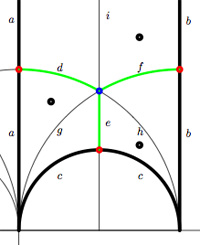$$\xymatrix{& & \rho \ar[lld]_d \ar[ld]^f \ar[rd]^e & \\ i \ar[rrd]_a & i+1 \ar[rd]^b & & \omega \ar[ld]^c \\ & & 0 \ar[uu]^h \ar@/^/[uu]^g \ar@/_/[uu]_i &}$$

In this case the super-potential coming from the triangulation is

$\Phi = -aid+agd-cge+che-bhf+bif$

and therefore we have a noncommutative algebra $\mathcal{U}(Q,\Phi)$ associated to this index 3 subgroup. Contrary to what I believed at the start of this series, the algebras one obtains in this way from dessins d’enfants are far from being Calabi-Yau (in whatever definition). For example, using a GAP-program written by Raf Bocklandt Ive checked that the growth rate of the above algebra is similar to that of $\mathbb{C}[x]$, so in this case $\mathcal{U}(Q,\Phi)$ can be viewed as a noncommutative curve (with singularities).

However, this is not the case for all such algebras. For example, the vacualgebra associated to the second index three subgroup (whose fundamental domain and quiver were depicted at the end of this post) has growth rate similar to that of $\mathbb{C} \langle x,y \rangle$…

I have an outlandish conjecture about the growth-behavior of all algebras $\mathcal{U}(Q,\Phi)$ coming from dessins d’enfants : the algebra sees what the monodromy representation of the dessin sees of the modular group (or of the third braid group).
I can make this more precise, but perhaps it is wiser to calculate one or two further examples…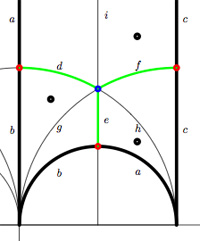We have associated to a subgroup of the modular group $PSL_2(\mathbb{Z})$ a quiver (that is, an oriented graph). For example, one verifies that the fundamental domain of the subgroup $\Gamma_0(2)$ (an index 3 subgroup) is depicted on the right by the region between the thick lines with the identification of edges as indicated. The associated quiver is then

$\xymatrix{i \ar[rr]^a \ar[dd]^b & & 1 \ar@/^/[ld]^h \ar@/_/[ld]_i \\ & \rho \ar@/^/[lu]^d \ar@/_/[lu]_e \ar[rd]^f & \\ 0 \ar[ru]^g & & i+1 \ar[uu]^c}$

The corresponding “dessin d’enfant” are the green edges in the picture. But, the red dot on the left boundary is identied with the red dot on the lower circular boundary, so the dessin of the modular subgroup $\Gamma_0(2)$ is

$\xymatrix{| \ar@{-}[r] & \bullet \ar@{-}@/^8ex/[r] \ar@{-}@/_8ex/[r] & -}$

Here, the three red dots (all of them even points in the Dedekind tessellation) give (after the identification) the two points indicated by a $\mid$ whereas the blue dot (an odd point in the tessellation) is depicted by a $\bullet$. There is another ‘quiver-like’ picture associated to this dessin, a quilt of the modular subgroup $\Gamma_0(2)$ as studied by John Conway and Tim Hsu.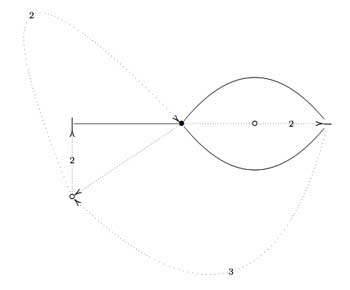On the left, a quilt-diagram copied from Hsu’s book Quilts : central extensions, braid actions, and finite groups, exercise 3.3.9. This ‘quiver’ has also 5 vertices and 7 arrows as our quiver above, so is there a connection?

A quilt is a gadget to study transitive permutation representations of the braid group $B_3$ (rather than its quotient, the modular group $PSL_2(\mathbb{Z}) = B_3/\langle Z \rangle$ where $\langle Z \rangle$ is the cyclic center of $B_3$. The $Z$-stabilizer subgroup of all elements in a transitive permutation representation of $B_3$ is the same and hence of the form $\langle Z^M \rangle$ where M is called the modulus of the representation. The arrow-data of a quilt, that is the direction of certain edges and their labeling with numbers from $\mathbb{Z}/M \mathbb{Z}$ (which have to satisfy some requirements, the flow rules, but more about that another time) encode the Z-action on the permutation representation. The dimension of the representation is $M \times k$ where $k$ is the number of half-edges in the dessin. In the above example, the modulus is 5 and the dessin has 3 (half)edges, so it depicts a 15-dimensional permutation representation of $B_3$.

If we forget the Z-action (that is, the arrow information), we get a permutation representation of the modular group (that is a dessin). So, if we delete the labels and directions on the edges we get what Hsu calls a modular quilt, that is, a picture consisting of thick edges (the dessin) together with dotted edges which are called the seams of the modular quilt. The modular quilt is merely another way to depict a fundamental domain of the corresponding subgroup of the modular group. For the above example, we have the indicated correspondences between the fundamental domain of $\Gamma_0(2)$ in the upper half-plane (on the left) and as a modular quilt (on the right)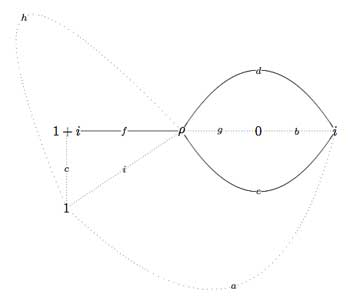That is, we can also get our quiver (or its opposite quiver) from the modular quilt by fixing the orientation of one 2-cell. For example, if we fix the orientation of the 2-cell $\vec{fch}$ we get our quiver back from the modular quilt$\xymatrix{i \ar[rr]^a \ar[dd]^b & & 1 \ar@/^/[ld]^h \ar@/_/[ld]_i \\ & \rho \ar@/^/[lu]^d \ar@/_/[lu]_e \ar[rd]^f & \\ 0 \ar[ru]^g & & i+1 \ar[uu]^c}$

This shows that the quiver (or its opposite) associated to a (conjugacy class of a) subgroup of $PSL_2(\mathbb{Z})$ does not depend on the choice of embedding of the dessin (or associated cuboid tree diagram) in the upper half-plane. For, one can get the modular quilt from the dessin by adding one extra vertex for every connected component of the complement of the dessin (in the example, the two vertices corresponding to 0 and 1) and drawing a triangulation from them (the dotted lines or ‘seams’).

In a couple of days I’ll be blogging for 4 years… and I’m in the process of resurrecting about 300 posts from a database-dump made in june. For example here’s my first post ever which is rather naive. This conversion program may last for a couple of weeks and I apologize for all unwanted pingbacks it will produce.

I’ll try to convert chunks of related posts in one go, so that I can at least give them correct self-references. Today’s work consisted in rewriting the posts of my virtual course, in march of this year, on dessins d’enfants and its connection to noncommutative geometry (a precursor of what Ive been blogging about recently). These posts were available through the PDF-archive but are from now on open to the internal search-function. Here are the internal links and a short description of their contents

Besides, I’ve added a few scattered old posts, many more to follow…

Here I will go over the last post at a more leisurely pace, focussing on a couple of far more trivial examples. Here’s the goal : we want to assign a quiver-superpotential to any subgroup of finite index of the modular group. So fix such a subgroup $\Gamma’$ of the modular group $\Gamma=PSL_2(\mathbb{Z})$ and consider the associated permutation representation of $\Gamma$ on the left-cosets $\Gamma/\Gamma’$. As $\Gamma \simeq C_2 \ast C_3$ this representation is determined by the action of the order 2 and order 3 generators of the modular group. There are a number of combinatorial gadgets to control the subgroup $\Gamma’$ and the associated permutation representation : (generalized) Farey symbols and dessins d’enfants.

Recall that the modular group acts on the upper-halfplane (the ‘hyperbolic plane’) by Moebius transformations, so to any subgroup $\Gamma’$ we can associate a fundamental domain for its restricted action. The dessins and the Farey symbols give us a particular choice of these fundamental domains. Let us consider the two most trivial subgroups of all : the modular group itself (so $\Gamma/\Gamma$ is just one element and therefore the associated permutation representation is just the trivial representation) and the unique index two subgroup $\Gamma_2$ (so there are two cosets $\Gamma/\Gamma_2$ and the order 2 generator interchanges these two while the order 3 generator acts trivially on them). The fundamental domains of $\Gamma$ (left) and $\Gamma_2$ (right) are depicted below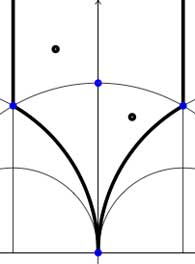In both cases the fundamental domain is bounded by the thick black (hyperbolic) edges. The left-domain consists of two hyperbolic triangles (the upper domain has $\infty$ as the third vertex) and the right-domain has 4 triangles. In general, if the subgroup $\Gamma’$ has index n, then its fundamental domain will consist of $2n$ hyperbolic triangles. Note that these triangles are part of the Dedekind tessellation so really depict the action of $PGL_2(\mathbb{Z}$ and any $\Gamma$-hyperbolic triangle consists of one black and one white triangle in Dedekind’s coloring. We will indicate the color of a triangle by a black circle if the corresponding triangle is black. Of course, the bounding edges of the fundamental domain need to be identified and the Farey symbol is a notation device to clarify this. The Farey symbols of the above domains are
$$\xymatrix{\infty \ar@{-}[r]_{\circ} & 0 \ar@{-}[r]_{\bullet} & \infty}$$ and $$\xymatrix{\infty \ar@{-}[r]_{\bullet} & 0 \ar@{-}[r]_{\bullet} & \infty}$$ respectively. In both cases this indicates that the two bounding edges on the left are to be identified as are the two bounding edges on the right (so, in particular, after identification $\infty$ coincides with $0$). Hence, after identification, the $\Gamma$ domain consists of two triangles on the vertices ${ 0,i,\rho }$ (where $\rho=e^{2 \pi i}{6}$) (the blue dots) sharing all three edges, the $\Gamma_2$ domain consists of 4 triangles on the 4 vertices ${ 0,i,\rho,\rho^2 }$ (the blue dots). In general we have three types of vertices : cusps (such as 0 or $\infty$), even vertices (such as $i$ where there are 4 hyperbolic edges in the Dedekind tessellation) and odd vertices (such as $\rho$ and $\rho^2$ where there are 6 hyperbolic edges in the tessellation).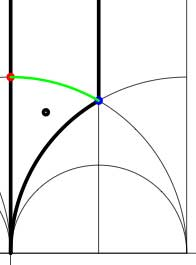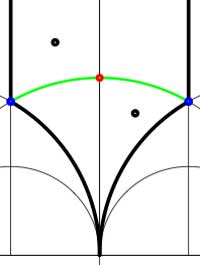Another combinatorial gadget assigned to the fundamental domain is the cuboid tree diagram or dessin. It consists of all odd and even vertices on the boundary of the domain, together with all odd and even vertices in the interior. These vertices are then connected with the hyperbolic edges connecting them. If we color the even vertices red and the odds blue we have the indicated dessins for our two examples (the green pictures). An half-edge is an edge connecting a red and a blue vertex in the dessin and we number all half-edges. So, the $\Gamma$-dessin has 1 half-edge whereas the $\Gamma_2$-dessin has two (in general, the number of these half-edges is equal to the index of the subgroup). Observe also that every triangle has exactly one half-edge as one of its three edges. The dessin gives all information to calculate the permutation representation on the coset-set $\Gamma/\Gamma’$ : the action of the order 2 generator of $\Gamma$ is given by taking for each internal red vertex the two-cycle $~(a,b)$ where a and b are the numbers of the two half-edges connected to the red vertex and the action of the order 3 generator is given by taking for every internal blue vertex the three cycle $~(c,d,e)$ where c, d and e are the numbers of the three half-edges connected to the blue vertex in counter-clockwise ordering. Our two examples above are a bit too simplistic to view this in action. There are no internal blue vertices, so the action of the order 3 generator is trivial in both cases. For $\Gamma$ there is also no red internal vertex, whence this is indeed the trivial representation whereas for $\Gamma_2$ there is one internal red vertex, so the action of the order 2 generator is given by $~(1,2)$, which is indeed the representation representation on $\Gamma/\Gamma_2$. In general, if the index of the subgroup $\Gamma’$ is n, then we call the subgroup of the symmetric group on n letters $S_n$ generated by the action-elements of the order 2 and order 3 generator the monodromy group of the permutation representation (or of the subgroup). In the trivial cases here, the monodromy groups are the trivial group (for $\Gamma$) and $C_2$ (for $\Gamma_2$).

As a safety-check let us work out all these concepts in the next simplest examples, those of some subgroups of index 3. Consider the Farey symbols

$$\xymatrix{\infty \ar@{-}[r]_{\circ} & 0 \ar@{-}[r]_{\circ} & 1 \ar@{-}[r]_{\circ} & \infty}$$ and
$$\xymatrix{\infty \ar@{-}[r]_{\circ} & 0 \ar@{-}[r]_{1} & 1 \ar@{-}[r]_{1} & \infty}$$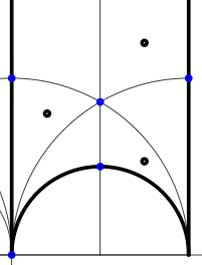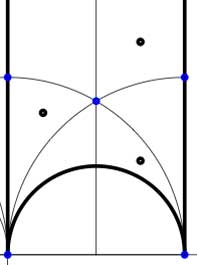In these cases the fundamental domain consists of 6 triangles with the indicated vertices (the blue dots). The distinction between the two is that in the first case, one identifies the two edges of the left, resp. bottom, resp. right boundary (so, in particular, 0,1 and $\infty$ are identified) whereas in the second one identifies the two edges of the left boundary and identifies the edges of the bottom with those of the right boundary (here, 0 is identified only with $\infty$ but also $1+i$ is indetified with $\frac{1}{2}+\frac{1}{2}i$).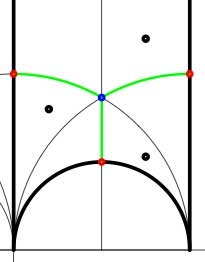In both cases the dessin seems to be the same (and given by the picture on the right). However, in the first case all three red vertices are distinct hence there are no internal red vertices in this case whereas in the second case we should identify the bottom and right-hand red vertex which then becomes an internal red vertex of the dessin!

Hence, if we order the three green half-edges 1,2,3 starting with the bottom one and counting counter-clockwise we see that in both cases the action of the order 3-generator of $\Gamma$ is given by the 3-cycle $~(1,2,3)$. The action of the order 2-generator is trivial in the first case, while given by the 2-cycle $~(1,2)$ in the second case. Therefore, the monodromy group is the cylic group $C_3$ in the first case and is the symmetric group $S_3$ in the second case.

Next time we will associate a quiver to these vertices and triangles as well as a cubic superpotential which will then allow us to define a noncommutative algebra associated to any subgroup of the modular group. The monodromy group of the situation will then reappear as a group of algebra-automorphisms of this noncommutative algebra!

Yesterday, Jan Stienstra gave a talk at theARTS entitled “Quivers, superpotentials and Dimer Models”. He started off by telling that the talk was based on a paper he put on the arXiv Hypergeometric Systems in two Variables, Quivers, Dimers and Dessins d’Enfants but that he was not going to say a thing about dessins but would rather focuss on the connection with superpotentials instead…pleasing some members of the public, while driving others to utter despair.

Anyway, it gave me the opportunity to figure out for myself what dessins might have to do with dimers, whathever these beasts are. Soon enough he put on a slide containing the definition of a dimer and from that moment on I was lost in my own thoughts… realizing that a dessin d’enfant had to be a dimer for the Dedekind tessellation of its associated Riemann surface!
and a few minutes later I could slap myself on the head for not having thought of this before :

There is a natural way to associate to a Farey symbol (aka a permutation representation of the modular group) a quiver and a superpotential (aka a necklace) defining (conjecturally) a Calabi-Yau algebra! Moreover, different embeddings of the cuboid tree diagrams in the hyperbolic plane may (again conjecturally) give rise to all sorts of arty-farty fanshi-wanshi dualities…

I’ll give here the details of the simplest example I worked out during the talk and will come back to general procedure later, when I’ve done a reference check. I don’t claim any originality here and probably all of this is contained in Stienstra’s paper or in some physics-paper, so if you know of a reference, please leave a comment. Okay, remember the Dedekind tessellation ?So, all hyperbolic triangles we will encounter below are colored black or white. Now, take a Farey symbol and consider its associated special polygon in the hyperbolic plane. If we start with the Farey symbol

$$\xymatrix{\infty \ar@{-}_{(1)}[r] & 0 \ar@{-}_{\bullet}[r] & 1 \ar@{-}_{(1)}[r] & \infty}$$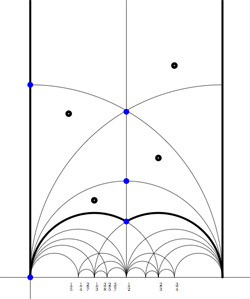we get the special polygonal region bounded by the thick edges, the vertical edges are identified as are the two bottom edges. Hence, this fundamental domain has 6 vertices (the 5 blue dots and the point at $i \infty$) and 8 hyperbolic triangles (4 colored black, indicated by a black dot, and 4 white ones).

Right, now let us associate a quiver to this triangulation (which embeds the quiver in the corresponding Riemann surface). The vertices of the triangulation are also the vertices of the quiver (so in our case we are going for a quiver with 6 vertices). Every hyperbolic edge in the triangulation gives one arrow in the quiver between the corresponding vertices. The orientation of the arrow is determined by the color of a triangle of which it is an edge : if the triangle is black, we run around its edges counter-clockwise and if the triangle is white we run over its edges clockwise (that is, the orientation of the arrow is independent of the choice of triangles to determine it). In our example, there is one arrows directed from the vertex at $i$ to the vertex at $0$, whether you use the black triangle on the left to determine the orientation or the white triangle on the right. If we do this for all edges in the triangulation we arrive at the quiver below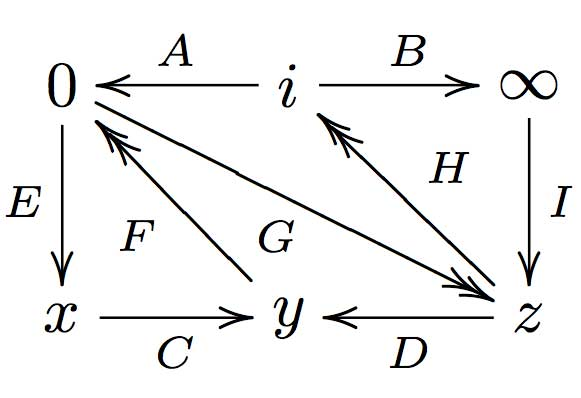where x,y and z are the three finite vertices on the $\frac{1}{2}$-axis from bottom to top and where I’ve used the physics-convention for double arrows, that is there are two F-arrows, two G-arrows and two H-arrows. Observe that the quiver is of Calabi-Yau type meaning that there are as much arrows coming into a vertex as there are arrows leaving the vertex.

Now that we have our quiver we determine the superpotential as follows. Fix an orientation on the Riemann surface (for example counter-clockwise) and sum over all black triangles the product of the edge-arrows counterclockwise MINUS sum over all white triangles
the product of the edge arrows counterclockwise. So, in our example we have the cubic superpotential

$IH’B+HAG+G’DF+FEC-BHI-H’G’A-GFD-CEF’$

From this we get the associated noncommutative algebra, which is the quotient of the path algebra of the above quiver modulo the following ‘commutativity relations’

$\begin{cases} GH &=G’H’ \\ IH’ &= IH \\ FE &= F’E \\ F’G’ &= FG \\ CF &= CF’ \\ EC &= GD \\ G’D &= EC \\ HA &= DF \\ DF’ &= H’A \\ AG &= BI \\ BI &= AG’ \end{cases}$

and morally this should be a Calabi-Yau algebra (( can someone who knows more about CYs verify this? )). This concludes the walk through of the procedure. Summarizing : to every Farey-symbol one associates a Calabi-Yau quiver and superpotential, possibly giving a Calabi-Yau algebra!